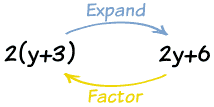### Factoring Review

 LEARNING OBJECTIVE:    BTEOTPSWBAT...... Chapter 5 Quadratic Functions ......... factor the GCF from an expression.- Use the difference of squares factoring RULE- basic trinomial factoring PART 1: 5-1 Transformations of Quadratic Functions

 BASIC FACTORINGPart 1:  Basics of Factoring - GCFPractice: Practice Factoring Linear BinomialsPart 2: Factor by Grouping (4 TERM FACTORING)Video: Factor by Grouping - BasicPart 3:  Difference of Squares (2 TERM FACTOR)Video: Factoring Difference of squaresPractice: Factoring Difference of SquaresVideo: Difference of Squares 2Practice: Difference of squares 2Video: Factoring to produce difference of squaresPractice: Factoring Difference of Squares 3 ADVANCED FACTORINGPart 1: Basic Trinomial Factoring (3 TERM)Some of you may have learned the x box methodPractice: Basic Trinomial Factoring PracticePart 2: Trinomials with a GCF (3 TERM)-Part 3:factor trinomials a not equal to 1 (3 TERM)Video: Factor Trinomials a not equal to 1Video 2: Factoring - trinomial a not equal 1Video 3: Factoring - trinomial a not equal to 1Video 4 - Factoring - Trinomial with a GCF, a doesn't equal 1Video 5 - Factoring - Trinomial with a GCF - a doesnt equal 1PRACTICE: Factoring All Trinomials!
Ċ
Brant Wilson,
Nov 5, 2014, 6:42 AM
Ċ
Brant Wilson,
Nov 5, 2014, 6:42 AM